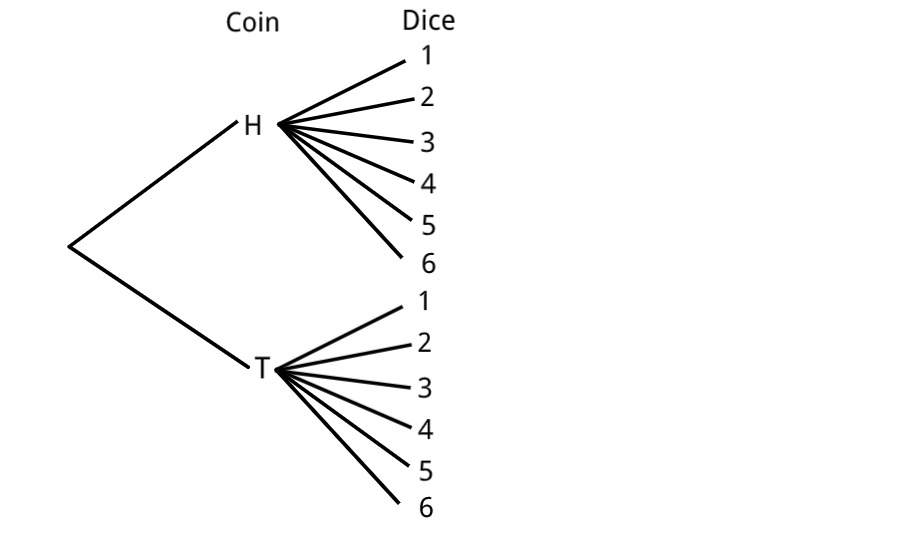# 3.2 Introducing the Addition Rule

Last updated: February 25th, 2020

Let's use again the example of flipping a coin and then throwing a dice. But now imagine we want to know the probability of getting Heads and $5$ or getting Tails and $2$.

Again, in a small example like this one we can solve it as before:

If $E=\{H5,T2\}$ then $P(E)=\dfrac{|E|}{|\Omega|}=\dfrac{2}{12}=\dfrac{1}{6}$, because it has equally likely results and $\Omega=\{H1,H2,H3,H4,H5,H6,T1,T2,T3,T4,T5,T6\}$.

But let's think about it in terms of our tree diagram:If we look closely, we are interested in two different branches, each of which has a probability of $\dfrac{1}{12}$.

So to calculate the probability of getting either of them, we can add the two probabilities: $P(H5 \ or \ T2)=P(H5)+P(T2)=\dfrac{1}{12}+\dfrac{1}{12}=\dfrac{2}{12}=\dfrac{1}{6}$.

In general, when we have two (or more) outcomes/events and we want the probability of either of them happening, we are going to add the individual probabilities.

Of course, this is going to be called the Addition Rule.

In terms of the tree diagram, we can say that the probability down the branches is the addition of the probabilities.

Again, one final and important remark: The Addition Rule has certain conditions that need to be met in order to use it, but we are going to dig deeper into that in future videos.